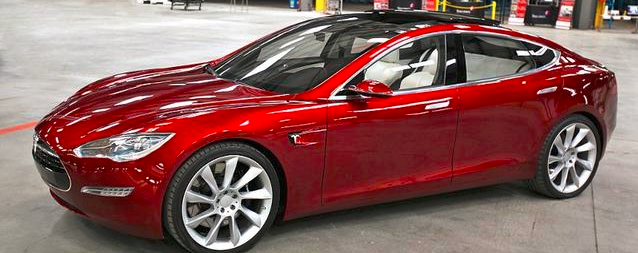ImageAI是一个Python库，旨在帮助开发人员构建具有自包含计算机视觉功能的应用程序和系统。

1. 安装Python 3.5.1或更高版本和pip

（如果您已经安装了Python 3.5.1或更高版本，请跳过本节）

2. 安装ImageAI依赖项

- Tensorflow

- Numpy

pip3 install numpy

- SciPy

pip3 install scipy

- OpenCV

pip3 install opencv-python

- Matplotlib

pip3 install matplotlib

- h5py

pip3 install h5py

- Keras

pip3 install keras

3. 安装ImageAI库

pip3 install https://github.com/OlafenwaMoses/ImageAI/raw/master/dist/imageai-1.0.2-py3-none-any.whl

5. 创建一个名称为python的文件（例如“FirstPrediction.py”），并将下面的代码写入其中。

from imageai.Prediction import ImagePrediction

import os

execution_path = os.getcwd()

prediction = ImagePrediction()

prediction.setModelTypeAsResNet()

prediction.setModelPath( execution_path + " esnet50_weights_tf_dim_ordering_tf_kernels.h5")

for index in range(len(predictions)):

print(predictions[index] + " : " + percentage_probabilities[index])

sample.jpgsports_car : 90.61029553413391

car_wheel : 5.9294357895851135

racer : 0.9972884319722652

convertible : 0.8457873947918415

grille : 0.581052340567112

代码说明

from imageai.Prediction import ImagePrediction

import os

execution_path = os.getcwd（）

prediction = ImagePrediction（）

prediction.setModelTypeAsResNet（）

prediction.setModelPath（execution_path +“ resnet50_weights_tf_dim_ordering_tf_kernels.h5”）

for index in range(len(predictions)):

print(predictions[index] + " : " + percentage_probabilities[index])